# A. No-go result for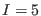inIn agreement with (86), the general solution to the equationcan be chosen to stop at antighost number(220)

where the components on the right-hand side of (181) are subject to the equations (68) and (66)-(67) for.

The pieceas solution to equation (68) forhas the general form expressed by (75) for, with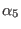from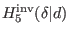. According to (81) at antighost number five, it follows thatis spanned by the generic representatives (82). Sinceshould effectively mix the BF and thetensor field sectors in order to produce cross-couplings and (82) involves only BF generators, it follows that one should retain from the basis elements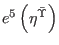only the objects containing at least one ghost from thetensor field sector, namely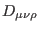or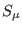. Recalling that we work precisely in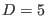, we obtain that the general solution to (68) forreduces to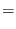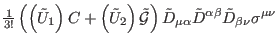(221)(222)

Each tilde object from the right-hand side of (182) means the Hodge dual of the corresponding non-tilde element, defined in general by formula ( 92). The elementsare dual toas in (82), with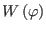respectively replaced by the smooth function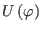depending only on the undifferentiated scalar field.

Introducing (182) in equation (66) forand recalling definitions (35)-(52), we obtain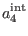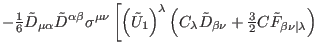(223)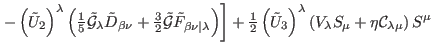(224)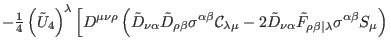(225)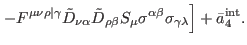(226)

In (183)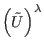are dual to (83 ), with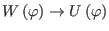. In addition,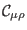is implicitly defined by formula (74) so it is a ghost field of pure ghost number one without definite symmetry/antisymmetry property,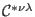is its associated antifield, defined such that the antibracketis equal to the `unit'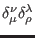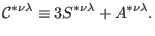(227)

The nonintegrated density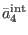stands for the solution to the homogeneous equation (68) for, showing thatcan be taken as a nontrivial element ofin pure ghost number equal to four.

At this stage it is useful to decomposeas a sum between two components(228)

whereis the solution to (68) forwhich is explicitly required by the consistency ofin antighost number three (ensures that (67) possesses solutions forwith respect to the terms from (183) containing the functions of the type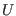) andsignifies the part of the solution to (68) forthat is independently consistent in antighost number three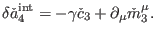(229)

Using definitions (35)-(52) and decomposition (185 ), by direct computation we obtain that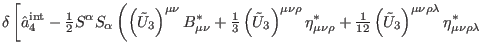(230)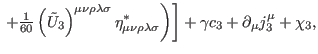(231)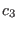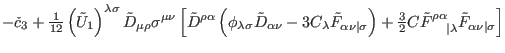(232)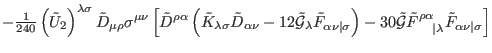(233)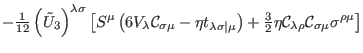(234)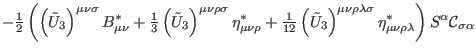(235)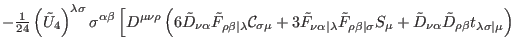(236)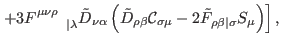(237)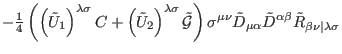(238)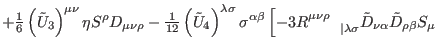(239)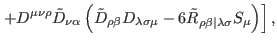(240)

andare some local currents. In (187)-(189)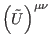anddenote the duals of (84) and (85) with. In addition,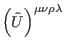represents the dual ofand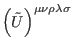the dual of. Inspecting (187), it follows that the consistency ofin antighost number three, namely the existence ofas solution to (67) for, requires the conditions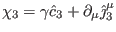(241)

and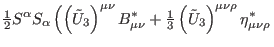(242)(243)

where we made the notations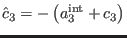and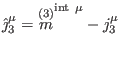. Nevertheless, from (189) it is obvious that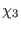is a nontrivial element fromin pure ghost number four, which does not reduce to a full divergence, and therefore (190) requires that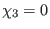, which further imply that all the functions of the typemust be some real constants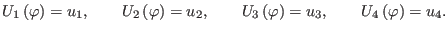(244)

Based on (192), it is clear thatgiven by ( 182) vanishes, and hence we can assume, without loss of nontrivial terms, that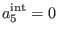(245)

in (181).

Ashkbiz Danehkar
2018-03-26